# Substitution method for solving differential equations

## Description of the method

The substitution method for solving differential equations is a method that is used to transform and manipulate differential equations and may help solve them.

The key idea is to replace the dependent variable or independent variable by a new variable that is expressed in terms of both of them.

### Zeroth order substitution

For a differential equation:$F(x,y,y',\dots,y^{(k)}) = 0$

a zeroth order substitution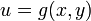$u = g(x,y)$ in place of$y$ works if we can rewrite the original condition as: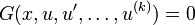$G(x,u,u',\dots,u^{(k)}) = 0$

After solving this differential equation relating$x$ and$u$, we plug back$u = g(x,y)$ to get relational solutions in terms of$x$ and$y$.

### Higher order substitutions

In some cases, we may substitute using a function not just of$x$ and$y$, but also of some of the derivatives of$y$. For instance, we may do a substitution$u = g(x,y,y', \dots, y^{(r)})$ for some function$g$ of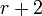$r + 2$ variables.

If we are doing this kind of substitution, then after solving the differential equation between$x$ and$u$, we need to come back and solve a differential equation between$x$ and$y$ by plugging$u = g(x,y,y',\dots,y^{(r)})$ in the solutions obtained. This is a differential equation of order$r$. In general, the order of the substitution determines the order of this second differential equation that we need to solve.

### Interchanging role of dependent and independent variable

In some cases, we may use the new variable to replace the independent variable rather than the dependent variable.

### Pure substitution

A substitution where the substituted variable is purely a function of$x$ or$y$ usually does not carry much power: it is equivalent to integration by substitution. The real power of the substitution method for differential equations (which cannot be done in integration alone) is when the function being substituted depends on both variables.

## Examples

### Zeroth-order substitution in first-order differential equation

Consider the differential equation:$y + x\frac{dy}{dx} = e^{x(1 + y)}$

Consider the substitution$u = xy$. Then,$du/dx = y + xdy/dx$ is the left side. The right side is$e^{x + u} = e^xe^u$. Thus, we get:$\frac{du}{dx} = e^xe^u$

The equation is now a separable differential equation, and can be solved that way:$\int e^{-u} \, du = \int e^x \, dx$

(Note that there are no stationary solutions).

We thus get, with freely varying parameter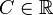$C \in \R$: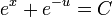$e^x + e^{-u} = C$

Plugging back$u = xy$, this gives:$e^x + e^{-xy} = C, \qquad C \in \R$

Solving for$y$ gives:$y = \frac{-1}{x} \ln \left(C - e^x \right), \qquad C \in \R$